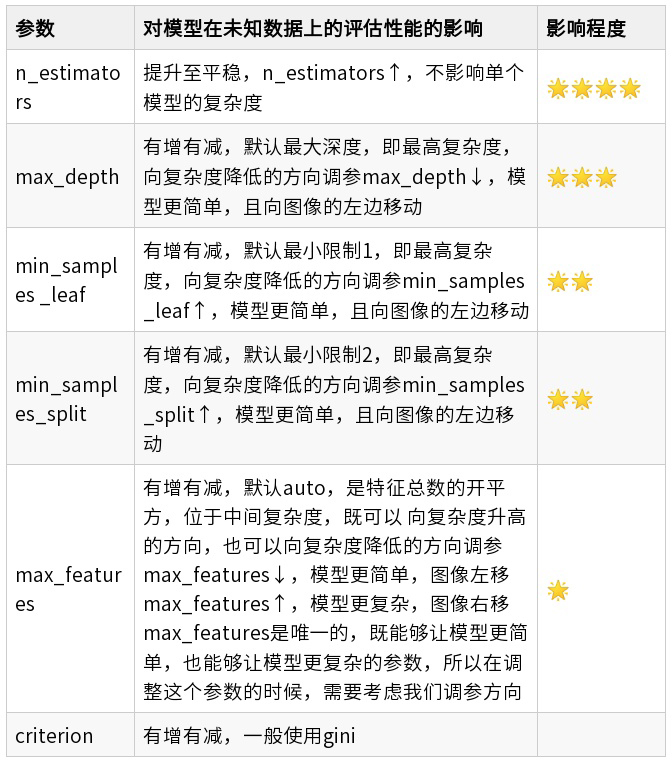##全国校区# 集成算法，随机森林回归模型2021-07-20

```RandomForestRegressor(n_estimators='warn',
criterion='mse',
max_depth=None,
min_samples_split=2,
min_samples_leaf=1,
min_weight_fraction_leaf=0.0,
max_features='auto',
max_leaf_nodes=None,
min_impurity_decrease=0.0,
min_impurity_split=None,
bootstrap=True,
oob_score=False,
n_jobs=None,
random_state=None,
verbose=0,
warm_start=False)```

# 一、重要参数，属性与接口

criterion

1. 输入"mse"使用均方误差mean squared error(MSE)，父节点和叶子节点之间的均方误差的差额将被用来作为特征选择的标准，这种方法通过使用叶子节点的均值来最小化L2损失。
2. 输入"friedman_mse"使用费尔德曼均方误差，这种指标使用弗里德曼针对潜在分枝中的问题改进后的均方误差。
3. 输入"mae"使用绝对平均误差MAE(mean absolute error)，这种指标使用叶节点的中值来最小化L1损失。

# 二、例子

```from sklearn.datasets import load_boston from sklearn.model_selection import cross_val_score from sklearn.ensemble import RandomForestRegressor
regressor = RandomForestRegressor(n_estimators=100,random_state=0)
cross_val_score(regressor, boston.data, boston.target, cv=10                ,scoring = "neg_mean_squared_error")
sorted(sklearn.metrics.SCORERS.keys())```

`import numpy as np import pandas as pd import matplotlib.pyplot as plt from sklearn.datasets import load_boston from sklearn.impute import SimpleImputer from sklearn.ensemble import RandomForestRegressor from sklearn.model_selection import cross_val_score`

```dataset = load_boston()
dataset.data.shape #总共506*13=6578个数据 X, y = dataset.data, dataset.target
n_samples = X.shape
n_features = X.shape```

`rng = np.random.RandomState(0) missing_rate = 0.5 n_missing_samples = int(np.floor(n_samples * n_features * missing_rate)) #np.floor向下取整，返回.0格式的浮点数 `

```missing_features_index = rng.randint(0,n_features,n_missing_samples)
missing_samples_index = rng.randint(0,n_samples,n_missing_samples) # missing_samples=rng.choice(dataset.data.shape,n_missing_samples,replace=False) X_missing = X.copy()
y_missing = y.copy()
X_missing[missing_samples, missing_features] = np.nan
X_missing = pd.DataFrame(X_missing) # 转换成DataFrame是为了后续⽅便各种操作， # numpy对矩阵的运算速度快，但是在索引等功能上却不如pandas来得好⽤ ```

```#使⽤均值进⾏填补 from sklearn.impute import SimpleImputer
imp_mean = SimpleImputer(missing_values=np.nan, strategy='mean')
X_missing_mean = imp_mean.fit_transform(X_missing) #使⽤0进⾏填补 imp_0 = SimpleImputer(missing_values=np.nan, strategy="constant",fill_value=0)
X_missing_0 = imp_0.fit_transform(X_missing)``````X_missing_reg = X_missing.copy()
sortindex = np.argsort(X_missing_reg.isnull().sum(axis=0)).values
for i in sortindex:
#构建我们的新特征矩阵和新标签     df = X_missing_reg
fillc = df.iloc[:,i]
df = pd.concat([df.iloc[:,df.columns != i],pd.DataFrame(y_full)],axis=1)
#在新特征矩阵中，对含有缺失值的列，进⾏0的填补     df_0 =SimpleImputer(missing_values=np.nan,
strategy='constant',fill_value=0).fit_transform(df)
#找出我们的训练集和测试集     Ytrain = fillc[fillc.notnull()]
Ytest = fillc[fillc.isnull()]
Xtrain = df_0[Ytrain.index,:]
Xtest = df_0[Ytest.index,:]
#⽤随机森林回归来填补缺失值     rfc = RandomForestRegressor(n_estimators=100)
rfc = rfc.fit(Xtrain, Ytrain)
Ypredict = rfc.predict(Xtest)
#将填补好的特征返回到我们的原始的特征矩阵中     X_missing_reg.loc[X_missing_reg.iloc[:,i].isnull(),i] = Ypredict```

```#对所有数据进⾏建模，取得MSE结果 X = [X_full,X_missing_mean,X_missing_0,X_missing_reg] mse = [] std = [] for x in X:
estimator = RandomForestRegressor(random_state=0, n_estimators=100)
scores =
cross_val_score(estimator,x,y_full,scoring='neg_mean_squared_error',
cv=5).mean()
mse.append(scores * -1)```

```x_labels = ['Full data',
'Zero Imputation',
'Mean Imputation',
'Regressor Imputation']
colors = ['r', 'g', 'b', 'orange']
plt.figure(figsize=(12, 6))
ax = plt.subplot(111) for i in np.arange(len(mse)):
ax.barh(i, mse[i],color=colors[i], alpha=0.6, align='center')

ax.set_title('Imputation Techniques with Boston Data')
ax.set_xlim(left=np.min(mse) * 0.9,right=np.max(mse) * 1.1)
ax.set_yticks(np.arange(len(mse)))
ax.set_xlabel('MSE')
ax.set_yticklabels(x_labels)
plt.show()```

# 三、随机森林调参OK